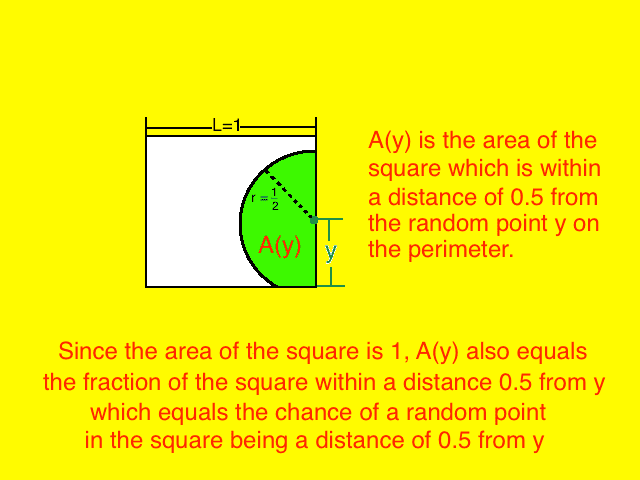# Probability of a point in a square being 0.5 from perimeter

Homework Helper

## Homework Statement

Consider a square of side length 1. Two points are chosen independently at random such that one is on the perimeter while the other is inside the square. Find the probability that the straight-line distance between the two points is at most 0.5.

2. The attempt at a solution
I assumed that I can just treat the point on the perimeter as if it's chosen at random along half of 1 side (from a corner to a midpoint) because no matter where the random point on the perimeter is chosen, the square can be reoriented (in our imagination) so that it lies on the same line segment (from a corner to the midpoint of a side). I feel I am justified in doing this because reorienting the square should not change the randomness of the point inside the square (because the point in the square is independently random, and also because all possible ways to reorient the square should be equally likely). Is this where I went wrong?

Let me proceed with this assumption.
Call the distance from the corner y. From the above assumption, y ranges from [0, 0.5]
Take a look at my drawing of the situation:I think dy/0.5 = 2dy would be the chance of the random point "being" y (or being within dy of y, or however you should think of it)

So I think the solution to the problem should be $\int\limits_0^{0.5} 2A(y)dy$

And finally, $A(y)=\int\limits_0^{y+0.5} \sqrt{0.25-(y-x)^2}dx$

This gives me an answer of π/8-1/12 ≈ 0.31 which seems reasonable, but is apparently
incorrect.

(It seems reasonable because at most A(y)=A(1/2)=pi/8≈0.4 and at least A(y)=A(0)=pi/16≈0.2 so the answer should be somewhere between 0.2 and 0.4)

I'm not sure what I did wrong.

## Answers and Replies

Homework Helper
Gold Member
at most 0.5.
Your sketch shows point "y" at one extreme, however, it can be anywhere from the corner to halfway "up." My take, I guarantee nothing.

Homework Helper
What is the probability that a point would fall into the quarter circle if the point on the perimeter were at the corner?
You seem to have worked out the half-circle problem for when the point falls in the middle.
The relative probability will be ##\int \int d(x,y) p(x) dx dy ##

Homework Helper
Dearly Missed

## Homework Statement

Consider a square of side length 1. Two points are chosen independently at random such that one is on the perimeter while the other is inside the square. Find the probability that the straight-line distance between the two points is at most 0.5.

2. The attempt at a solution
I assumed that I can just treat the point on the perimeter as if it's chosen at random along half of 1 side (from a corner to a midpoint) because no matter where the random point on the perimeter is chosen, the square can be reoriented (in our imagination) so that it lies on the same line segment (from a corner to the midpoint of a side). I feel I am justified in doing this because reorienting the square should not change the randomness of the point inside the square (because the point in the square is independently random, and also because all possible ways to reorient the square should be equally likely). Is this where I went wrong?

Let me proceed with this assumption.
Call the distance from the corner y. From the above assumption, y ranges from [0, 0.5]
Take a look at my drawing of the situation:

View attachment 78854

I think dy/0.5 = 2dy would be the chance of the random point "being" y (or being within dy of y, or however you should think of it)

So I think the solution to the problem should be $\int\limits_0^{0.5} 2A(y)dy$

And finally, $A(y)=\int\limits_0^{y+0.5} \sqrt{0.25-(y-x)^2}dx$

This gives me an answer of π/8-1/12 ≈ 0.31 which seems reasonable, but is apparently
incorrect.

(It seems reasonable because at most A(y)=A(1/2)=pi/8≈0.4 and at least A(y)=A(0)=pi/16≈0.2 so the answer should be somewhere between 0.2 and 0.4)

I'm not sure what I did wrong.

I get your answer as well; why do you think it is wrong?

•Nathanael
Homework Helper
Hello Nate/Richard :)

Did what you did, found what you found ##{\pi\over 8} - {1\over 12}##.

Corroborated with a rude numerical simulation (16000 x 3 excel numbers :) , 12 times; found 0.30905 +/- 0.00071)

So here's a second one who agrees with you.
What tells you it's wrong ?

•Nathanael
Homework Helper
Oh, wow... The problem asked, what is the probability that the distance will be at least 0.5...arghuhghgrumbles... 13/12 - pi/8

I am very sorry for wasting all your time!

Homework Helper
Dearly Missed
Oh, wow... The problem asked, what is the probability that the distance will be at least 0.5...arghuhghgrumbles... 13/12 - pi/8

I am very sorry for wasting all your time!

No time wasted at all. Computing 1 - P(d < .05) is by far the easiest way to compute P(d >= 0.5). This is clear at once if you refer back to your diagram.